# PSEB 9th Class Maths Solutions Chapter 7 Triangles Ex 7.1

Punjab State Board PSEB 9th Class Maths Book Solutions Chapter 7 Triangles Ex 7.1 Textbook Exercise Questions and Answers.

## PSEB Solutions for Class 9 Maths Chapter 7 Triangles Ex 7.1

Question 1.
In quadrilateral ACBD. AC = AD and AB bisects ∠ A (see the given figure). Show that ∆ ABC ≅ ∆ ABD. What can you say about BC and BD?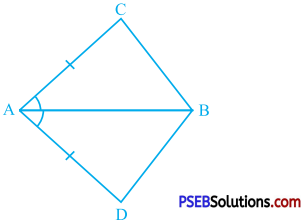In ∆ ABC and ∆ ABD,
∠ BAC = ∠ BAD (AB bisects ∠ A)
AB = AB (Common)
∴ ∆ ABC ≅ ∆ ABD (SAS rule)
∴ BC = BD (CPCT)
Thus, BC and BD are equal.Question 2.
ABCD is a quadrilateral in which AD = BC and ∠ DAB = ∠ CBA (see the given figure). Prove that (i) ∆ ABD ≅ ∆ BAC, (ii) BD = AC and (iii) ∠ ABD = ∠ BAC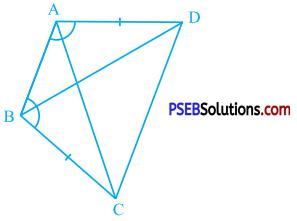In ∆ ABD and ∆ BAC,
∠ DAB = ∠ CBA (Given)
AB = BA (Common)
∴ ∆ ABD ≅ ∆ BAC (SAS rule)
∴ BD = AC (CPCT)
∴ ∠ ABD = ∠ BAC (CPCT)

Question 3.
AD and BC are equal perpendiculars to a line segment AB (see the given figure). Show that CD bisects AB.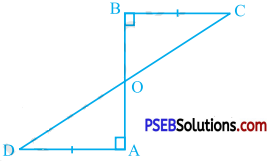AD and BC are equal perpendiculars to line segment AB.
∴ AD = BC and ∠ OAD = ∠ OBC = 90°.
Now, in ∆ ADO and ∆ BCO,
∠ AOD = ∠ BOC (Vertically opposite angles)
∴ ∆ ADO ≅ ∆ BCO (AAS rule)
∴ OA = OB (CPCT)
CD intersects AB at O and OA = OB.
Hence, CD bisects AB.Question 4.
l and m are two parallel lines intersected by another pair of parallel lines p and q (see the given figure). Show that:
∆ ABC ≅ ∆ CDA.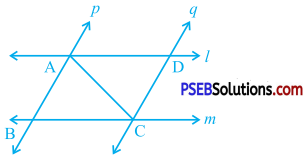l || m and AC is their transversal.
∴ ∠ BCA = ∠ DAC (Alternate angles)
p l| q and AC is their transversal.
∴ ∠ BAC = ∠ DCA (Alternate angles)
Now, in ∆ ABC and ∆ CDA,
∠ BCA = ∠ DAC
∠ BAC = ∠ DCA
AC = CA (Common)
∴ ∆ ABC ≅ ∆ CDA (ASA rule)

Question 5.
Ray l is the bisector of an angle ∠ A and B is any point on l. BP and BQ are perpendiculars from B to the arms of ∠ A (see the given figure). Show that:
(i) ∆ APB ≅ ∆ AQB
(ii) BP = BQ or B is equidistant from the arms of ∠ A.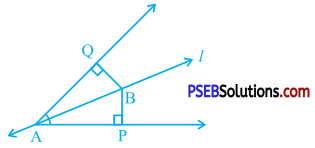l is the bisector of ∠ PAQ and B is any point on l.
∴ ∠ PAB = ∠ QAB
BP and BQ are perpendiculars from B to AP and AQ.
∴ ∠ BPA = ∠ BQA = 90°.
Now, in ∆ APB and ∆ AQB,
∠ PAB = ∠ QAB
∠ BPA = ∠ BQA
AB = AB (Common)
∴ ∆ APB ≅ ∆ AQB (AAS rule)
∴ BP = BQ (CPCT)
BP and BQ are perpendiculars from B to arms AP and AQ of ∠ A.
∴ BP is the distance of B from AP and BQ is the distance of B from AQ.
Thus, B is equidistant from the arms of ∠ A.Question 6.
In the given figure, AC = AE, AB = AD and ∠ BAD = ∠ EAC. Show that BC = DE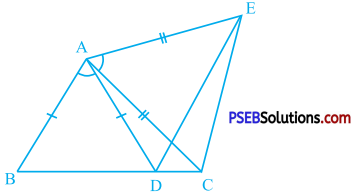∴ ∠ BAD + ∠ DAC = ∠ EAC + ∠ DAC
∴ ∠ BAC = ∠ DAE (Adjacent angles)
Now, in ∆ BAC and ∆ DAE,
AC = AE (Given)
∠ BAC = ∠ DAE
∴ ∆ BAC ≅ ∆ DAE (SAS rule)
∴ BC = DE (CPCT)

Question 7.
AB is a line segment and P is its midpoint. D and E are points on the same side of AB such that ∠ BAD = ∠ ABE and ∠ EPA = ∠ DPB (see the given figure). Show that:
(i) ∆ DAP ≅ ∆ EBP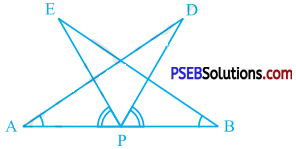∴ ∠ PAD = ∠PBE (∵ P lies on AB.)
∠ EPA = ∠ DPB
∴ ∠ EPA + ∠ EPD = ∠ DPB + ∠ EPD
∴ ∠ APD = ∠ BPE (Adjacent angles)
P is the midpoint of AB.
∴ AP = BP
Now, in ∆ DAP and ∆ EBP
∠ APD = ∠ BPE
AP = BP
∴ ∆ DAP ≅ ∆ EBP (ASA rule)Question 8.
In right triangle ABC, right angled at C, M is the midpoint of hypotenuse AB. C is joined to M and produced to a point D such that DM = CM. Point D is joined to point B (see the given figure). Show that:
(i) ∆ AMC ≅ ∆ BMD
(ii) ∠ DBC is a right angle
(iii) ∆ DBC ≅ ∆ ACB
(iv) CM = $$\frac{1}{2}$$ AB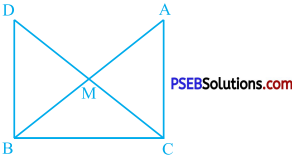In ∆ AMC and ∆ BMD,
AM = BM (∵ M is the midpoint of AB.)
CM = DM (Given)
∠ AMC = ∠ BMD (Vertically opposite angles)
∴ By SAS rule, ∆ AMC ≅ ∆ BMD [Result (i)]
∴ ∠ MCA = ∠ MDB (CPCT)
∠ MCA and ∠ MDB are alternate angles formed by transversal CD of lines AC and BD and they are equal.
∴ AC || BD
Now, ∠ DBC and ∠ ACB are interior angles on the same side of transversal BC of AC || BD.
∴ ∠ DBC + ∠ ACB = 180°
∴ ∠ DBC + 90° = 180° (Given : ∠ C = 90°)
∴ ∠ DBC = 90°
Thus, ∠ DBC is a right angle. [Result (ii)]
Now, ∆ AMC ≅ ∆ BMD
∴ AC = BD
In ∆ DBC and ∆ ACB,
BD = CA
∠ DBC = ∠ ACB (Right angles)
BC = CB (Common)
∴ ∆ DBC ≅ ∆ ACB [Result (iii)]
∴ DC = AB (CPCT)
DM = CM and M lies on line’ segment CD.
∴ DC = 2 CM
∴ AB = 2CM
∴ $$\frac{1}{2}$$AB = CM
∴ CM = $$\frac{1}{2}$$AB